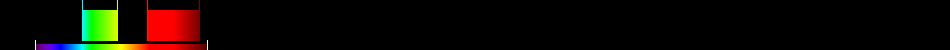# Information for Sensor and Index

## Formula

General Formula $\frac{{\mathrm{NDVI}}+0,5}{|{\mathrm{NDVI}}+0,5|}·\sqrt{|\left({\mathrm{NDVI}}\right)+0,5|}$ $\frac{\left(\frac{{\mathrm{red}}-{\mathrm{green}}}{{\mathrm{red}}+{\mathrm{green}}}\right)+0,5}{|\left(\frac{{\mathrm{red}}-{\mathrm{green}}}{{\mathrm{red}}+{\mathrm{green}}}\right)+0,5|}·\sqrt{|\left(\left(\frac{{\mathrm{red}}-{\mathrm{green}}}{{\mathrm{red}}+{\mathrm{green}}}\right)\right)+0,5|}$ Automatic

## Sensor

Name CBERS-1/CBERS-2 8 450-890 2.7-260 0 Brazil and China 2003-10-21 CBERS-2 is identical to CBERS-1 and was launched 2003-10-21 yes

## Index

Name Corrected Transformed Vegetation Index CTVI $\frac{{\mathrm{NDVI}}+0,5}{|{\mathrm{NDVI}}+0,5|}·\sqrt{|\left({\mathrm{NDVI}}\right)+0,5|}$ 490:570,640:760 Derived from specific sensor formula

## References